or# Straight Lines NCERT Solutions : Class 11 Maths

## NCERT Solutions for Class 11 Maths : Straight LinesFilter
Filters :
Classes
•
•
•
•
•
•
•
Chapters
•
•
•
•
•
•
•
•
•
•
• 6 More
Exercises
•
•
•
•
•
•
•

Doubtnut one of the best online education platform provides free NCERT Solutions of Maths for Class 11 Straight Lines which are solved by our maths experts as per the NCERT (CBSE) guidelines. We provide the solutions as video solutions in which concepts are also explained along with answer which will help students while learning , preparing for exams and in doing homework. These Solutions will help to revise complete syllabus and score more marks in examinations.

Get here free, accurate and comprehensive NCERT Solutions for Class 11 Maths Straight Lines which have been reviewed by our maths counsellors as per the latest edition following up the CBSE guidelines. We provide video solutions in which solutions to all the questions of NCERT Class 11 Maths textbook are explained in step by step and detailed way.

## The Topics of the Chapter Straight Lines are :

DISTANCE BETWEEN PARALLEL LINES

DISTANCE OF A POINT FROM A LINE

DIFFERENT FORMS OF THE EQUATION OF STRAIGHT LINE

QUESTIONS

ANGLE BETWEEN TWO LINES

LINE PARALLEL & PERPENDICULAR TO A GIVEN LINE

POINT OF INTERSECTION OF TWO LINES

DIFFERENT FORMS OF LINE

EQUATION OF THE LINES PARALLEL TO THE COORDINATE AXES

CONDITION OF CONCURRENCY OF TWO LINES

AREA OF A TRIANGLE

DEFINITION OF STRAIGHT LINE

SECTION FORMULA

POSITION OF A POINT RELATIVE TO A PLANE

INTERCEPT OF A LINE ON THE AXES

SHIFTING OF ORIGIN

It contains these exercises along with solved examples. All the exercises are solved in the video. Select the exercise to view the solutions exercisewise of the Chapter Straight Lines:

## NCERT Solutions Class 11 Maths Chapter Straight Lines Exercises:

We have covered all the exercises and also Solved examples in the videos. Along with the practise exercise Students should also practise solved examples to clear the concepts of the Straight Lines. If incase you have any doubt you can watch the solutions for the given questions.Watch the solutions for the given questions in which it is also explained in the video steps to solve the questions along with answers.

In this chapter, we will concentrate on Straight Lines. Here, we will cover all important topics like slope of a line, angle between two lines, collinearity of three points, various forms of the equation of a line, horizontal and vertical lines, and so on. Here, we will get to know about general equation of a line as well. Doubtnut has provided lots of different examples and that too with exercises so that the students do not find any sort of problem in getting the accurate solutions.

It is seen that NCERT textbooks prove to be useful for the students when it comes to clearing the basic concepts. NCERT textbooks are important for the CBSE board Students But it has also been found that it fails to provide enough solved examples to attain an in depth understanding that leads to a lot of problem in scoring good marks in the exams. Moreover, Math is a subject that requires consistent practice to master the complex mathematical concepts. So, a student who is not able to clear their queries would surely find it much difficult in approaching tricky or complex questions.

The expert tutors of Doubtnut understand the problems faced by the students in attempting the tricky questions. So, in order to make things less complicated for the students, the subject matter experts have curated the study material in the form interactive video tutorials and easy downloadable pdfs. These study materials help students to equip with all the knowledge in the simplest manner. Each and every problem have been thoroughly explained in a lucid and coherent language. where they find all the right idea as to how to attempt it without any difficulties at all. The study materials are provided free of cost where the students do not have to pay anything for it. So, the students are able to clear all their confusions in a comprehensive manner and thus leading to scoring higher marks in the examinations. Moreover, the NCERT solutions provided by Doubtnut are helpful in quick revision for preparation of competitive exams like IIT JEE Mains and IIT JEE Advanced.

## Topics included in NCERT Class 11 Maths Solutions Chapter 10 Straight Lines

### Introduction

From our earlier classes, we have learned about two-dimensional coordinate geometry. In this chapter, we will learn more about coordinate geometry where it will cover, ‘straight line’ which happens to be the simplest geometric figure. We will mainly focus on how to represent the line algebraically and for this, ‘slope’ is very important.

### Slope of a Line

Two angles are formed by a line in a coordinate plane which are supplementary.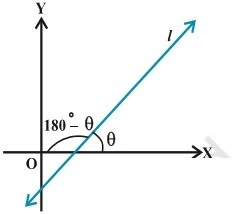The angle θ which is made by the line ‘l’ and that too with positive direction of x-axis along with anti-clockwise measurement is known as the inclination of the line. So, in the above figure, 0° ≤ θ ≤ 180°

Definition: If θ happens to be the inclination of a line, ‘l’, then tan θ is known as the gradient or slope of the line, ‘l.’

The slope of a line whose inclination is 900 is not defined.

‘m’ is denoted for the slope of a line.

So, m = tan θ, θ ≠ 90°

We can get a clear observation that the zero is the slope of x-axis while the slope of y-axis is not defined.

### Collinearity of three points

We are familiar that slopes of two parallel lines are equal. It is to be noted that two lines will coincide in case they have the same slope that passes through a common point.

Here in the below figure, A, B and C are three points in the XY-plane. They lie on a line where you can find three points are collinear. Here slope of AB = slope of BC.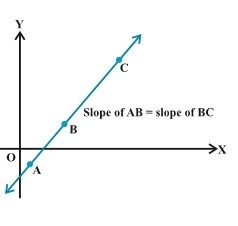Example:  Three points P (h, k), Q (x1, y1) and R (x2, y2) lie on a line. Show that

(h – x1) (y2 – y1) = (k – y1) (x2 – x1).

Solution: We find that P,Q and R are collinear.

So, we have,

Slope of PQ = Slope of QR,

i.e.  y1 - k = y2 - y1

x1- h   x2 –x1

or    k –y1 = y2 – y1

h – x1     x2 – x1

or       (h – x1) (y2 – y1) = (k – y1) (x2 – x1)

### Various Forms of the Equation of a Line

Have you ever wondered as to how you can prove that a given point lies on the given line? Here, we should have a different condition on the points that are lying on the line. Let’s take for an example that P (x, y) is an arbitrary point in the XY-plane and L is the given line. In order to get the equation of L, it is important to construct a condition or statement for the point P that is true. Here P should be on L.  But when it comes to the condition where P is not on L, then it would be false. So, here we are going to discuss the equation of a line under different conditions.

### Horizontal and vertical lines

A horizontal line, ‘L’ is at a distance, ‘a’ from the x-axis. Here, a or –a would be the ordinate of every point lying on the line [Figure 10.11 (a)]

So, here the equation of line will be either y = a or y = -a.

In the same way, at a distance, ‘b’ from the y-axis for the equation of a vertical line will be either x = b or x = -b [Figure 10.11 (b)]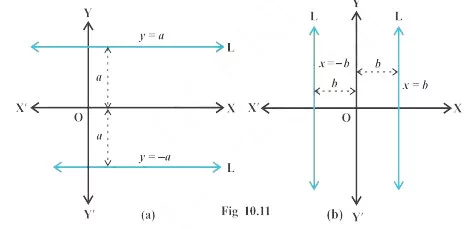Example: Find out the equation of the line through (-2, 3) when the slope is -4

Solution: In this case, we find that m =-4 and the given point (x0, y0) is (-2, 3)

With the use of slope-intercept form formula,

y -3 = -4 (x +2) or

4x + y + 5 = 0 which happens to be the required equation.

### General Equation of a Line

We know that the general equation of first degree in two variables is Ax + By + C = 0, where A, B and C are real constants such that A and B are not zero simultaneously. Graph of the equation Ax + By + C = 0 is always a straight line.

So, in this case any equation of the form Ax + By + C = 0, where A and B are not zero simultaneously is called general linear equation or general equation of a line.

### Different forms of Ax + By + C = 0

It is important to know that it is possible to reduce the general equation of a line into various forms with the help of the following procedures:

(a) Slope-intercept form: If B ≠ 0, then Ax + By + C = 0 can be written as

y = Ax – C  or y = mx + c               ………….    (1)

B      B

where m = A and c =

B             B

We know that Equation (1) is the slope-intercept form of the equation of a line

whose slope is A and y-intercept is  C

B                                 B

Here, if B =0, then x= C which is a vertical line whose slope is undefined and

A

x intercepts is – C

A

(b) Intercept form:  If C ≠ 0, then Ax + By + C = 0 can be written as

x + y = 1 or x + y = 1                           …………….  (2)

C -  C         a     b

A    B

Where we can find a = C and b = - C

A              5 B

We have found that equation (2) is intercept form of the equation of a line whose

x-intercept is – C and y-intercept is - C

A                                  B

Here, if C =0 then Ax + By + C = 0 can be written as Ax + By = 0, which is a line passing through the origin and, therefore, has zero intercepts on the axes.

(c) Normal form: In the same way, when it comes to its normal form of Ax + By + C = 0, it is x cos ω + y sin ω = p

Example: Find the equation of a line perpendicular to the line x -2y + 3 = 0 and passing through              the point (1, – 2).

Solution:  Here the given line is x – 2y + 3 = 0.  This can be written as:

y = 1 x + 3                                ………..  (1)

2       2

Here, slope of the line (1) happens to be m1 = 1. So, slope of the perpendicular to line (1) is

m2 = - 1 = -2

m1    2

Equation of the line with slope – 2 and passing through the point (1, – 2) is

y - (- 2) = -2(x - 1) or y = -2x which happens to be the required equation.

### Other distances

It is also important to know the right idea as to how to find the distance of a point from a line and also the distance between the two parallel lines. Here, we will have a look at an example to get the right idea about it.

Example: Find the distance of the line 4x – y = 0 from the point P (4, 1) measured along the line making an angle of 135° with the positive x-axis.

Solution:  Given line is 4x – y = 0 ... (1)

In order to find the distance of the line (1) from the point P (4, 1) along another line, we have to find the point of intersection of both the lines. For this purpose, we will first find the equation of the second line (look at the figure).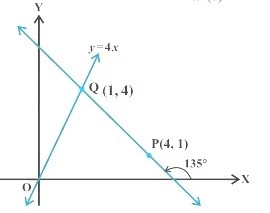Slope of second line is tan 135° = –1.

Equation of the line with slope – 1 through the point P (4, 1) is

y – 1 = – 1 (x – 4) or x + y – 5 = 0 ... (2)

Solving (1) and (2), we get x = 1 and y = 4 so that point of intersection of the two lines is Q (1, 4). Now, distance of line (1) from the point P (4, 1) along the line (2)

= the distance between the points P (4, 1) and Q (1, 4).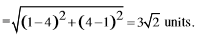## Role of Doubtnut for Class 11 Maths students

The role played by the team of the experts of Doubtnut is really worthwhile. It has been possible to boost the confidence level of the students. The students get the clear idea on how to solve the different questions without taking much time at all by following the Class 11 Maths NCERT Solutions Chapter 10. So, this not only makes them fast but also helps to achieve success without any sort of problem. Some of the advantages of learning via Doubtnut are:

• Doubtnut’s online study materials is structured in a step by step manner that helps students to get the proper idea on how to attempt even the trickiest questions effortlessly. One can also find important tips on how to come up with the perfect solution by working on it in the best way.
• The online study materials can also be accessed by the students no matter where they are. In other words, it proves to be quite flexible where they can study according to their preference. Being easily downloadable in the form of pdf, it serves to be of much use to them in clearing their concepts. So, students can really benefit out of it that would help in clearing their queries related to any subjects.
• Lots of examples and exercises can be seen that proves to be an added advantage to the students. So, getting all the right idea on how to work on the different problems becomes possible by opting for the perfect online video tutorials. It would help the students to develop a liking for the subject where they would also be able to find it quite interesting. The different exercises found in the study materials can also help to practice it on regular basis without fail.
• The study material is provided at zero cost. The students do not have to pay any subscription fee, any course fee, or any amount to access the quality study material.